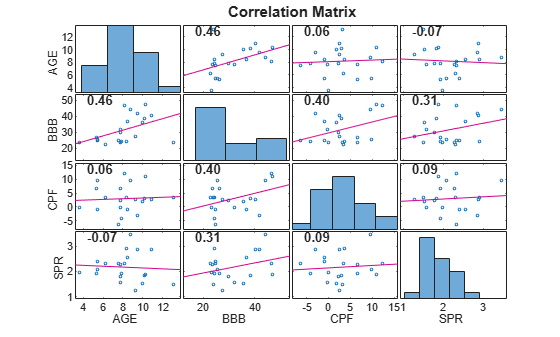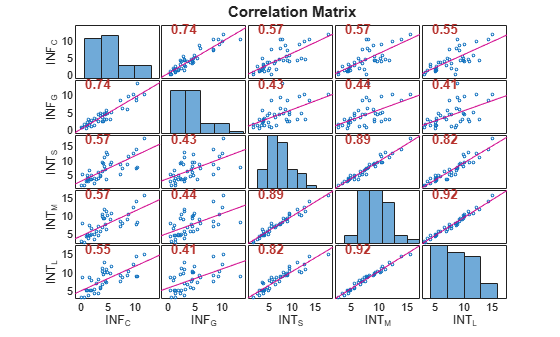# corrplot

Plot variable correlations

## Syntax

``corrplot(X)``
``corrplot(X,Name,Value)``
``R = corrplot(___)``
``````[R,PValue] = corrplot(___)``````
``corrplot(ax,___)``
``````[R,PValue,H] = corrplot(___)``````

## Description

example

``corrplot(X)` creates a matrix of plots showing correlations among pairs of variables in `X`. Histograms of the variables appear along the matrix diagonal; scatter plots of variable pairs appear in the off diagonal. The slopes of the least-squares reference lines in the scatter plots are equal to the displayed correlation coefficients.`

example

``corrplot(X,Name,Value)` uses additional options specified by one or more name-value pair arguments. For example, `corrplot(X,'type','Spearman','testR','on')` computes Spearman’s rank correlation coefficient and tests for significant correlation coefficients.`

example

``R = corrplot(___)` returns the correlation matrix of `X` displayed in the plots using any of the input argument combinations in the previous syntaxes.`

example

``````[R,PValue] = corrplot(___)``` additionally returns the p-values resulting from the test of the null hypothesis of no correlation against the alternative of a nonzero correlation. Elements of `PValue` correspond to the elements of `R`.```
````corrplot(ax,___)` plots on the axes specified by `ax` instead of the current axes (`gca`). `ax` can precede any of the input argument combinations in the previous syntaxes.```
``````[R,PValue,H] = corrplot(___)``` additionally returns handles to plotted graphics objects. Use elements of `h` to modify properties of the plot after you create it.```

## Examples

collapse all

Plot correlations between multiple time series.

`load Data_Canada`

Plot the Pearson's linear correlation coefficients between all pairs of variables.

`corrplot(DataTable)`The correlation plot shows that the short-term, medium-term, and long-term interest rates are highly correlated.

To examine the timestamp of a datum, enter `gname(dates)` into the Command Window, and the software presents an interactive cross hair over the plot. To expose the timestamp of a datum, click it using the cross hair.

Plot Kendall's rank correlations between multiple time series. Conduct a hypothesis test to determine which correlations are significantly different from zero.

`load Data_Canada`

Plot the Kendall's rank correlation coefficients between all pairs of variables. Specify a hypothesis test to determine which correlations are significantly different from zero.

`corrplot(DataTable,'type','Kendall','testR','on')`The correlation coefficients highlighted in red indicate which pairs of variables have correlations significantly different from zero. For these time series, all pairs of variables have correlations significantly different from zero.

Test for correlations greater than zero between multiple time series.

`load Data_Canada`

Return the pairwise Pearson's correlations and corresponding p-values for testing the null hypothesis of no correlation against the right-tailed alternative that the correlations are greater than zero.

`[R,PValue] = corrplot(DataTable,'tail','right');``PValue`
```PValue = 5×5 1.0000 0.0000 0.0000 0.0000 0.0000 0.0000 1.0000 0.0000 0.0000 0.0001 0.0000 0.0000 1.0000 0.0000 0.0000 0.0000 0.0000 0.0000 1.0000 0.0000 0.0000 0.0001 0.0000 0.0000 1.0000 ```

The output `PValue` has pairwise p-values all less than the default 0.05 significance level, indicating that all pairs of variables have correlation significantly greater than zero.

## Input Arguments

collapse all

Time series data, specified as a `numObs`-by-`numVars` numeric matrix, table, or timetable. `X` consists of `numObs` observations on `numVars` numeric variables.

Data Types: `double` | `table` | `timetable`

Axes on which to plot, specified as an `Axes` object.

By default, `corrplot` plots to the current axes (`gca`).

`corrplot` does not support `UIAxes` targets.

### Name-Value Pair Arguments

Specify optional comma-separated pairs of `Name,Value` arguments. `Name` is the argument name and `Value` is the corresponding value. `Name` must appear inside quotes. You can specify several name and value pair arguments in any order as `Name1,Value1,...,NameN,ValueN`.

Example: `'tails','right','alpha',0.1` specifies right-tailed tests at the 0.1 significance level

Correlation coefficient to compute, specified as the comma-separated pair consisting of `'type'` and one of the following:

 `'Pearson'` Pearson’s linear correlation coefficient `'Kendall'` Kendall’s rank correlation coefficient (τ) `'Spearman'` Spearman’s rank correlation coefficient (ρ)

Example: `'type','Kendall'`

Data Types: `char` | `string`

Option for handling rows with `NaN` values, specified as the comma-separated pair consisting of `'rows'` and one of the following:

 `'all'` Use all rows, regardless of `NaN`s. `'complete'` Use only rows with no `NaN`s. `'pairwise'` Use rows with no `NaN`s in column i or j to compute R(i,j).

Example: `'rows','complete'`

Data Types: `char` | `string`

Alternative hypothesis (Ha) used to compute the p-values, specified as the comma-separated pair consisting of `'tail'` and one of the following:

 `'both'` Ha: Correlation is not zero. `'right'` Ha: Correlation is greater than zero. `'left'` Ha: Correlation is less than zero.

Example: `'tail','left'`

Data Types: `char` | `string`

Variable names to be used in the plots, specified as the comma-separated pair consisting of `'varNames'` and a string vector or cell array of character vectors with `numVars` names. All variable names are truncated to the first five characters.

• If `X` is a matrix, the default variable names are `{'var1','var2',...}`.

• If `X` is a table or timetable, the default variable names are `X.Properties.VariableNames`.

Example: `'varNames',{'CPF','AGE','BBD'}`

Data Types: `cell` | `string`

Significance tests indicator for whether or not to test for significant correlations, specified as the comma-separated pair consisting of `'testR'` and one of `'off'` or `'on'`. If you specify the value `'on'`, significant correlations are highlighted in red in the correlation matrix plot.

Example: `'testR','on'`

Data Types: `char` | `string`

Significance level for tests of correlation, specified as a scalar between 0 and 1.

Example: `'alpha',0.01`

Data Types: `double`

## Output Arguments

collapse all

Correlations between pairs of variables in `X` that are displayed in the plots, returned as a `numVars`-by-`numVars` matrix.

p-values corresponding to significance tests on the elements of `R`, returned as a `numVars`-by-`numVars` matrix. The p-values are used to test the hypothesis of no correlation against the alternative of nonzero correlation.

Handles to plotted graphics objects, returned as a `numVars`-by-`numVars` graphics array. `H` contains unique plot identifiers, which you can use to query or modify properties of the plot.

## Tips

• The option `'rows','pairwise'`, which is the default, can return a correlation matrix that is not positive definite. The `'complete'` option always returns a positive-definite matrix, but in general the estimates are based on fewer observations.

• Use `gname` to identify points in the plots.

## Algorithms

The software computes:

• p-values for Pearson’s correlation by transforming the correlation to create a t-statistic with `numObs` – 2 degrees of freedom. The transformation is exact when `X` is normal.

• p-values for Kendall’s and Spearman’s rank correlations using either the exact permutation distributions (for small sample sizes) or large-sample approximations.

• p-values for two-tailed tests by doubling the more significant of the two one-tailed p-values.